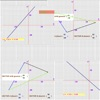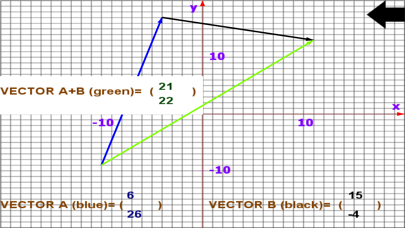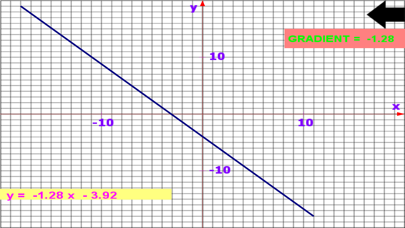## 2D Math Graph - Lumos Educational App StorePrice -Free

#### DESCRIPTION:

Learn about simple linear equation, y=mx+c. Draw, add 2d vectors automatically. This app will help beginners in graphical methods understand the linear equation, y=mx+c. Learn about cartesian plane, linear graphs and vectors simply by just a swipe. Find the relationship between lines, gradients, y-intercept, linear equations and vectors by drawing a graph with your finger while this app does the rest. No input of values is necessary. This is an automatic graph app. Math Graph app – you draw graph, it works out the equation for you. Not only does the app work out the y = mx + c equation

#### OVERVIEW:

2D Math Graph is a free educational mobile app By Harun Kimani.It helps students practice the following standards .

This page not only allows students and teachers download 2D Math Graph but also find engaging Sample Questions, Videos, Pins, Worksheets, Books related to the following topics.

Developer URL: NA

Software Version: 1.0.1

Category: Education

Release Date: 2016-09-02T10:57:58Z#### Are you the Developer?

Rate this App?
1.0

1 Ratings & 1 Reviews

5
0
0
4
0
0
3
0
0
2
0
0
1
100
1EdSearch WebSearch